# Tag: Manin

MUBs (for Mutually Unbiased Bases) are quite popular at the moment. Kea is running a mini-series Mutual Unbias as is Carl Brannen. Further, the Perimeter Institute has a good website for its seminars where they offer streaming video (I like their MacromediaFlash format giving video and slides/blackboard shots simultaneously, in distinct windows) including a talk on MUBs (as well as an old talk by Wootters).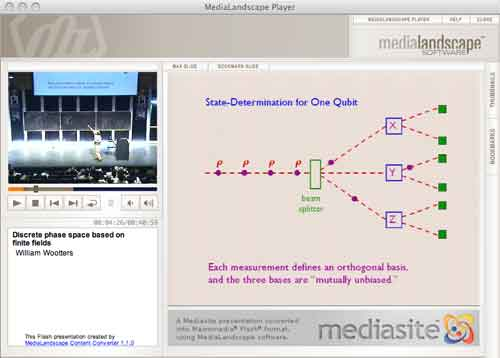So what are MUBs to mathematicians? Recall that a d-state quantum system is just the vectorspace $\mathbb{C}^d$ equipped with the usual Hermitian inproduct $\vec{v}.\vec{w} = \sum \overline{v_i} w_i$. An observable $E$ is a choice of orthonormal basis ${ \vec{e_i} }$ consisting of eigenvectors of the self-adjoint matrix $E$. $E$ together with another observable $F$ (with orthonormal basis ${ \vec{f_j} }$) are said to be mutally unbiased if the norms of all inproducts $\vec{f_j}.\vec{e_i}$ are equal to $1/\sqrt{d}$. This definition extends to a collection of pairwise mutually unbiased observables. In a d-state quantum system there can be at most d+1 mutually unbiased bases and such a collection of observables is then called a MUB of the system. Using properties of finite fields one has shown that MUBs exists whenever d is a prime-power. On the other hand, existence of a MUB for d=6 still seems to be open…

The King’s Problem (( actually a misnomer, it’s more the poor physicists’ problem… )) is the following : A physicist is trapped on an island ruled by a mean
king who promises to set her free if she can give him the answer to the following puzzle. The
physicist is asked to prepare a d−state quantum system in any state of her choosing and give it
to the king, who measures one of several mutually unbiased observables on it. Following this, the physicist is allowed to make a control measurement
on the system, as well as any other systems it may have been coupled to in the preparation
phase. The king then reveals which observable he measured and the physicist is required
to predict correctly all the eigenvalues he found.

The Solution to the King’s problem in prime power dimension by P. K. Aravind, say for $d=p^k$, consists in taking a system of k object qupits (when $p=2l+1$ one qupit is a spin l particle) which she will give to the King together with k ancilla qupits that she retains in her possession. These 2k qupits are diligently entangled and prepared is a well chosen state. The final step in finding a suitable state is the solution to a pure combinatorial problem :

She must use the numbers 1 to d to form $d^2$ ordered sets of d+1 numbers each, with repetitions of numbers within a set allowed, such that any two sets have exactly one identical number in the same place in both. Here’s an example of 16 such strings for d=4 :

11432, 12341, 13214, 14123, 21324, 22413, 23142, 24231, 31243, 32134, 33421, 34312, 41111, 42222, 43333, 44444

Here again, finite fields are used in the solution. When $d=p^k$, identify the elements of $\mathbb{F}_{p^k}$ with the numbers from 1 to d in some fixed way. Then, the $d^2$ of number-strings are found as follows : let $k_0,k_1 \in \mathbb{F}_{p^k}$ and take as the first 2 numbers the ones corresponding to these field-elements. The remaning d-2 numbers in the string are those corresponding to the field element $k_m$ (with $2 \leq m \leq d$) determined from $k_0,k_1$ by the equation

$k_m = l_{m} * k_0+k_1$

where $l_i$ is the field-element corresponding to the integer i ($l_1$ corresponds to the zero element). It is easy to see that these $d^2$ strings satisfy the conditions of the combinatorial problem. Indeed, any two of its digits determine $k_0,k_1$ (and hence the whole string) as it follows from
$k_m = l_m k_0 + k_1$ and $k_r = l_r k_0 + k_1$ that $k_0 = \frac{k_m-k_r}{l_m-l_r}$.

In the special case when d=3 (that is, one spin 1 particle is given to the King), we recover the tetracode : the nine codewords

0000, 0+++, 0—, +0+-, ++-0, +-0+, -0-+, -+0-, –+0

encode the strings (with +=1,-=2,0=3)

3333, 3111, 3222, 1312, 1123, 1231, 2321, 2132, 2213

Here a list of saved pdf-files of previous NeverEndingBooks-posts on geometry in reverse chronological order.

Yesterday, Yuri Manin and Matilde Marcolli arXived their paper
follow-up of their previous paper Continued fractions, modular symbols, and non-commutative geometry.
They motivate the title of the recent paper by :

In
[MaMar2](http://www.arxiv.org/abs/hep-th/0201036), these and similar
results were put in connection with the so called “holography”
principle in modern theoretical physics. According to this principle,
quantum field theory on a space may be faithfully reflected by an
appropriate theory on the boundary of this space. When this boundary,
rather than the interior, is interpreted as our observable
space‚Äìtime, one can proclaim that the ancient Plato’s cave metaphor
is resuscitated in this sophisticated guise. This metaphor motivated
the title of the present paper.

Here’s a layout of
Plato’s cave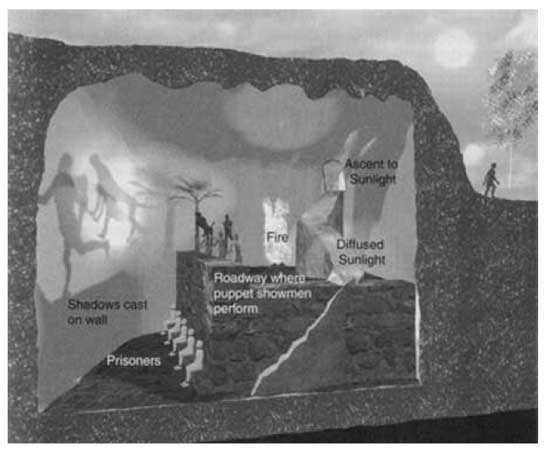Imagine prisoners, who have been chained since childhood deep inside an
cave: not only are their limbs immobilized by the chains; their heads
are chained as well, so that their gaze is fixed on a wall.
Behind
the prisoners is an enormous fire, and between the fire and the
prisoners is a raised walkway, along which statues of various animals,
plants, and other things are carried by people. The statues cast shadows
on the wall, and the prisoners watch these shadows. When one of the
statue-carriers speaks, an echo against the wall causes the prisoners to
believe that the words come from the shadows.
The prisoners
engage in what appears to us to be a game: naming the shapes as they
come by. This, however, is the only reality that they know, even though
they are seeing merely shadows of images. They are thus conditioned to
judge the quality of one another by their skill in quickly naming the
shapes and dislike those who begin to play poorly.
Suppose a
prisoner is released and compelled to stand up and turn around. At that
moment his eyes will be blinded by the firelight, and the shapes passing
will appear less real than their shadows.

Right, now how
does the Manin-Marcolli cave look? My best guess is : like this
picture, taken from Curt McMullen’s Gallery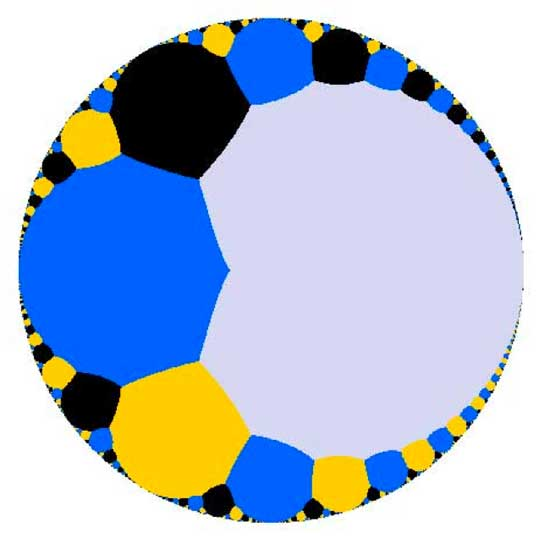Imagine
this as the top view of a spherical cave. M&M are imprisoned in the
cave, their heads chained preventing them from looking up and see the
ceiling (where $PSL_2(\mathbb{Z})$ (or a cofinite subgroup of
it) is acting on the upper-half plane via
Moebius-transformations ). All they can see is the circular exit of the
cave. They want to understand the complex picture going on over their
heads from the only things they can observe, that is the action of
(subgroups of) the modular group on the cave-exit
$\mathbb{P}^1(\mathbb{R})$. Now, the part of it consisting
of orbits of cusps
$\mathbb{P}^1(\mathbb{Q})$ has a nice algebraic geometric
description, but orbits of irrational points cannot be handled by
algebraic geometry as the action of $PSL_2(\mathbb{Z})$ is
highly non-discrete as illustrated by another picture from McMullen’s
gallerydepicting the ill behaved topology of the action on the bottom real
axis. Still, noncommutative _differential_ geometry is pretty good at
handling such ill behaved quotient spaces and it turns out that as a
noncommutative space, this quotient
$\mathbb{P}^1(\mathbb{R})/PSL_2(\mathbb{Z})$ is rich enough
to recover many important aspects of the classical theory of modular
curves. Hence, they reverse the usual NCG-picture of interpreting
commutative objects as shadows of noncommutative ones. They study the
$\mathbb{P}^1(\mathbb{R})/PSL_2(\mathbb{Z})$ of a classical
commutative object, the quotient of the action of the modular group (or
a cofinite subgroup of it) on the upper half-plane.

In our
noncommutative geometry course we have already
seen this noncommutative shadow in action (though at a very basic
level). Remember that we first described the group-structure of the
modular group $PSL_2(\mathbb{Z}) = C_2 \ast C_3$ via the
classical method of groups acting on trees. In particular, we
considered the tree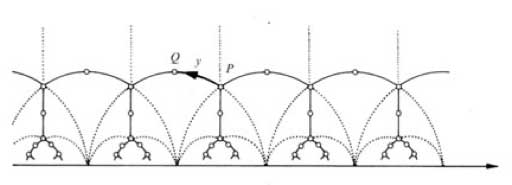and
calculated the stabilizers of the end points of its fundamental domain
(the thick circular edge). But
later we were able to give a
much shorter proof (due to Roger Alperin) by looking only at the action
of $PSL_2(\mathbb{Z})$ on the irrational real numbers (the
noncommutative shadow). Needless to say that the results obtained by
Manin and Marcolli from staring at their noncommutative shadow are a lot
more intriguing…

Who was the first mathematician to give a slide show talk? I don’t have the
definite answer to this question, but would like to offer a strong
candidate :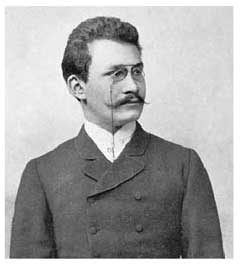Hermann Minkowski gave the talk “Zur Geometrie der Zahlen” (On the
geometry of numbers) before the third ICM in 1904 in Heidelberg and even
the title page of his paper in the proceedings indicates that he did
present his talk using slides (Mit Projektionsbildern auf einer
Doppeltafel)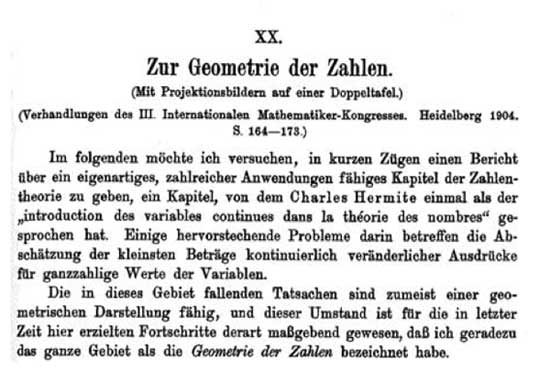Seven
of these eight slides would be hard to improve using LaTeX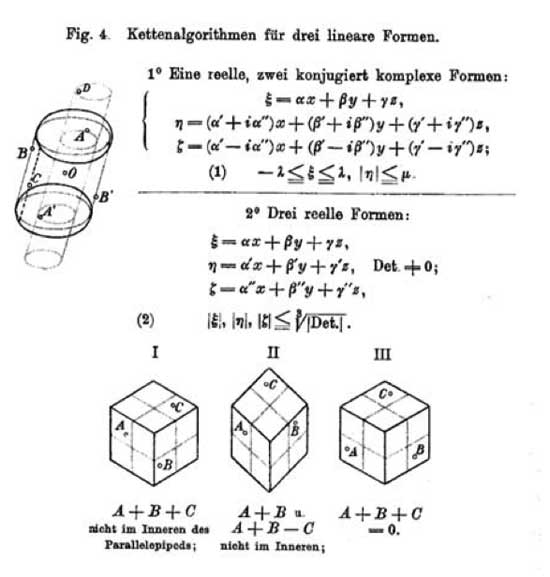What concerns
us today is the worst of all slides, the seventh, where Minkowski tries
to depict his famous questionmark function $?(x)$, sometimes also called
the _devil’s staircase_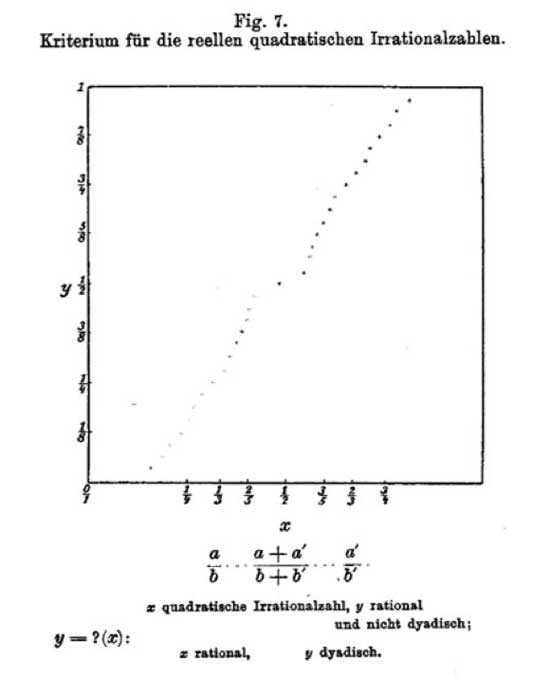The devil’s
staircase is a fractal curve and can be viewed as a mirror (taking a
point on the horizontal axis to the point on the vertical axis through
the function value) having magical simplifying properties : – it takes
rational numbers to _dyadic numbers_, that is those of the form
$n.2^{-m}$ with $n,m \in \mathbb{Z}$. – it takes quadratic
_irrational_ numbers to rational numbers. So, iterating this
mirror-procedure, the devil’s staircase is a device solving the main
problem of Greek Mathematics : which lengths can be constructed using
ruler and compass? These _constructible numbers_ are precisely those
real numbers which become after a finite number of devil-mirrors a
dyadic number. The proofs of these facts are not very difficult but
they involve a piece of long-forgotten mathematical technology :
_continued fractions_. By repeted approximations using the
floor-function (the largest natural number less than or equal to the real
number), every positive real number can be written as

$a = a_0 + \frac{1}{a_1 + \frac{1}{a_2 + \frac{1}{a_3 + \frac{1}{\dots}}}}$

with all $a_i$ natural numbers. So, let us just denote from now on this
continued fraction of a by the expression

$a = \langle a_0;a_1,a_2,a_3,\dots \rangle$

Clearly, a is a rational number if
(and also if but this requires a small argument using the Euclidian
algorithm) the above description has a tail of zeroes at the end and
(slightly more difficult) $a$ is a real quadratic irrational number
(that is, an element of a quadratic extension field
$\mathbb{Q}\sqrt{n}$) if and only if the continued fraction-expression
has a periodic tail. There is a lot more to say about
continued-fraction expressions and I’ll do that in another
‘virtual-course-post’ (those prepended with a (c): sign). For the
impatient let me just say that two real numbers will lie in the same
$GL_2(\mathbb{Z})$-orbit (under the action via Moebius-transformations)
if and only if their continued fraction expressions have the same tails
eventually (which has applications in noncommutative geometry as in the
work of Manin and Marcolli but maybe I’ll come to this in the (c):
posts).

Right, now we can define the mysterious devil-stair function
$?(x)$. If a is in the real interval $[0,1]$ and if $a \in \mathbb{Q}$ then $a = \langle 0;a_1,a_2,\dots,a_n,0,0,\dots \rangle$ and we define $?(a) = 2 \sum_{k=1}^{n} (-1)^k 2^{-(a_1+a_2+\dots+a_k)}$ and if a is irrational with continued
fraction expression $a = \langle 0;a_1,a_2,a_3,\dots \rangle$, then

$?(a) = 2 \sum_{k=1}^{\infty} (-1)^{k+1} 2^{-(a_1+a_2+\dots+a_k)}$

A
perhaps easier description is that with the above continued-fraction
expression, the _binary_ expansion of $?(a)$ has the following form

$?(a) = 0,0 \dots 01 \dots 1 0 \dots 0 1 \dots 1 0 \dots 0 1 \dots 1 0 \dots$

where the first batch of zeroes after the comma has length
$a_1-1$, the first batch of ones has length $a_2$ the next batch of
zeroes length $a_3$ and so on.

It is a pleasant exercise to verify that
this function does indeed have the properties we claimed before. A
recent incarnation of the question mark function is in Conway’s game of
_contorted fractions_. A typical position consists of a finite number of
boxed real numbers, for example the position might be

$\boxed{\pi} + \boxed{\sqrt{2}} + \boxed{1728} + \boxed{-\frac{1}{3}}$

The Rules of the game are : (1) Both
players L and R take turns modifying just one of the numbers such that
the denominator becomes strictly smaller (irrational numbers are
supposed to have $\infty$ as their ‘denominator’). And if the boxed
number is already an integer, then its absolute value must decrease.
(2) Left must always _decrease_ the value of the boxed number, Right
must always increase it. (3) The first player unable to move looses
the game. To decide who wins a particular game, one needs to compute
the value of a position $\boxed{x}$ according to the rules of
combinatorial game theory (see for example the marvelous series of four
books Winning Ways for your Mathematical Plays. It turns out that this CG-value is no other than $?(x)$
… And, Conway has a much improved depiction of the devil-staircase in
his book On Numbers And Games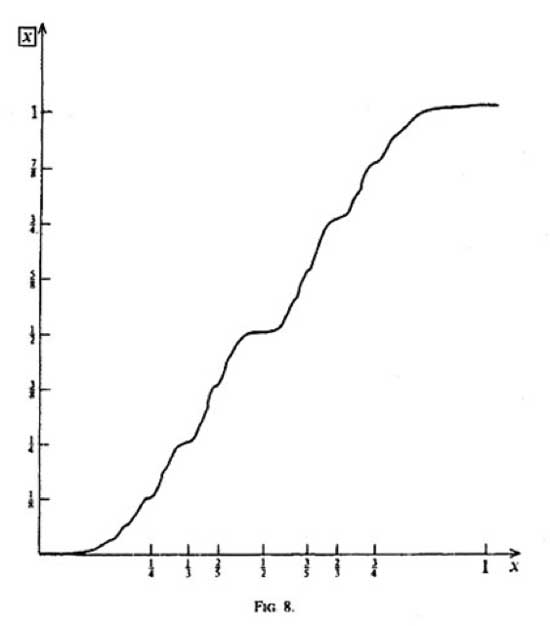Now
that my non-geometry
post
string-coffee-table post
which in turn is available through a
paper

The little
this : if you have a situation where a discrete group is acting with a
bad orbit-space (for example, $GL_2(\mathbb{Z})$ acting on the whole
complex-plane, rather than just the upper half plane) you can associate
to this a $C^*$-algebra and study invariants of it and interprete them
example is the one I mentioned and where the additional noncommutative
points (coming from the orbits on the real axis) seem to contain a lot
of modular information as clarified by work of Manin&Marcolli and
Zagier. Probably the best introduction into Connes-style
non-commutative geometry
from this perspective are the Lecture on
Arithmetic Noncommutative Geometry
by Matilde Marcolli. To
algebraists : this trick is very similar to looking at the
skew-group algebra $\mathbb{C}[x_1,\ldots,x_n] * G$ if
you want to study the _orbifold_ for a finite group action on affine
space. But as algebraist we have to stick to affine varieties and
polynomials so we can only deal with the case of a finite group,
analysts can be sloppier in their functions, so they can also do
something when the group is infinite.

By the way, the
skew-group algebra idea is also why non-commutative algebraic
geometry
enters string-theory via the link with orbifolds. The
easiest (and best understood) example is that of Kleinian singularities.
The best introduction to this idea is via the Representations
of quivers, preprojective algebras and deformations of quotient
singularities
notes by Bill Crawley-Boevey.

Artin-style non-commutative geometry aka
non-commutative projective geometry originated from the
work of Artin-Tate-Van den Bergh (in the west) and Odeskii-Feigin (in
the east) to understand Sklyanin algebras associated to elliptic curves
and automorphisms via ‘geometric’ objects such as point- (and
fat-point-) modules, line-modules and the like. An excellent survey
paper on low dimensional non-commutative projective geometry is Non-commutative curves and surfaces by Toby
Stafford and
Michel Van den Bergh
. The best introduction is the (also
neverending…) book-project Non-
commutative algebraic geometry
by Paul Smith who
maintains a
noncommutative geometry and algebra resource page
page (which is

Non-geometry
started with the seminal paper ‘Algebra extensions and
nonsingularity’, J. Amer. Math. Soc. 8 (1995), 251-289 by Joachim
Cuntz
and Daniel Quillen but which is not available online. An
online introduction is Noncommutative smooth
spaces
by Kontsevich and Rosenberg. Surely, different people have
different motivations to study non-geometry. I assume Cuntz got
interested because inductive limits of separable algebras are quasi-free
(aka formally smooth aka qurves). Kontsevich and Soibelman want to study
morphisms and deformations of $A_{\infty}$-categories as they explain in
their recent
paper
. My own motivation to be interested in non-geometry is the
hope that in the next decades one will discover new exciting connections
between finite groups, algebraic curves and arithmetic groups (monstrous
moonshine
being the first, and still not entirely understood,
instance of this). Part of the problem is that these three topics seem
to be quite different, yet by taking group-algebras of finite or
arithmetic groups and coordinate rings of affine smooth curves they all
turn out to be quasi-free algebras, so perhaps non-geometry is the
unifying theory behind these seemingly unrelated topics.